# IBPS PO Mains 2018 – Reasoning Ability Questions Day-26

Dear Readers, Bank Exam Race for the Year 2018 is already started, To enrich your preparation here we have providing new series of Practice Questions on Reasoning Ability – Section. Candidates those who are preparing for IBPS PO Mains 2018 Exams can practice these questions daily and make your preparation effective.

[WpProQuiz 4272]

Direction (1-5): Read the following information carefully and answer the questions given below.

Eight persons J, K, L, M, N, O, P and Q are sitting in the outer square table of the given diagram below. Four of them are sitting in the corners of the table and four of them are sitting in the middle of the sides. The persons sitting in the corners of the table are facing away from the centre and the persons sitting in the middle of the sides are facing centre of the table.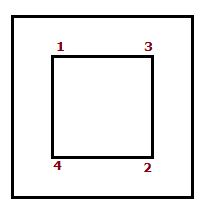Two persons are sitting between P and J, who does not sit in the middle of the sides. Q sits second to the left of N, who is an immediate neighbour of P.L sits second to the right of K. K is not an immediate neighbour of J.M does not sit opposite to K and not an immediate neighbour of L.

Then all the persons in the outer table are moved to the inner table. The four corners of the table are numbered as 1,2,3 and 4. They throw two dies probably and moved from the outer table to the inner table according to the given conditions given below.

i) If the sum of the two dies is even number, then the person moves to the corner-2 and faces the same direction as before.

ii) If the sum of the two dies is perfect square, then the person moves to the corner-4 and faces the direction opposite to the before.

iii) If the sum of the two dies is odd number, then the person moves to the corner-1 and faces the same direction as before.

iv) If the sum of the dies is multiple of 5, then the person moves to the middle of the sides between the corners-2 and 3 and faces opposite direction as before.

Note: Give first preference to the perfect square of a number and multiple of 5 and then go for an even and odd numbers.

Throws:

The one who sits to the immediate left of Q throws the dies and the sum is 7.

The one who faces L throws the dies and the sum is 4.

The one who sits second to the right of J throws the dies and the sum is 6.

The one who sits opposite to J throws the dies and the sum is 10.

After these processes, answer the questions given below.

1) Who among the following persons sits at corner-2?

a)P

b) Q

c) J

d) M

e) K

2) Four of the following five are alike in a certain way and hence form a group. Which one of the following that does not belong to the group?

a) P

b) Q

c) J

d) N

e) M

3) How many persons are sitting between M and K, when counted from left of K in the same table?

a) No one

b) One

c) Two

d) Three

e) More than three

4) Which of the following pairs represents the persons facing centre of the table in the inner table?

a) P, L

b)O, K

c) L, N

d)Q, L

e) N, M

5) If J is related to K and L is related P in a certain way. Then, M is related to which of the following?

a) J

b) O

c) L

d) N

e) Q

Directions (6-10): In the following questions, the symbols @, #, \$, % and & are used with the following meaning as illustrated below.

[email protected]’ means ‘P is neither greater than nor equal to Q’

‘P%Q’ means ‘P is neither equal to nor smaller than Q’

‘P&Q’ means ‘P is neither smaller than nor greater than Q’

‘P\$Q’ means ‘P is not smaller than Q’

‘P#Q’ means ‘P is not greater than Q’

Now in each of the following questions assuming the given statement to be true, find which of the conclusions given below them is/are definitely true and give your answer accordingly.

6) Statements:W#N&K%S; A%D\$K.

Conclusions:

I. A\$S

II. D%W

III. W&D

a)Only I is true

b) Only I and III are true

c) Only II is true

d) Only either II or III is true

e) All I, II and III are true

7) Statements:F%R\$E&D;P#R#G.

Conclusions:

I.G%F

II. D#G

a) Only II is true

b) Only I is true

c) Only II and either II or III are true

d) Only I and III are true

e) None is true

8) Statements:

C&H#N; J%M\$C&K.

Conclusions:

[email protected]

II. N\$K

III. M%N

a) Only I is true

b) Only either I or III are true

c) Only I and II are true

d) All I, II and III are true

e) Only III is true

9) Statements:

J\$U&K#E; [email protected]#V.

Conclusions:

I. M\$J

II. V%U

III. U#V

a) None is true

b) Only II is true

c) Only III is true

d) Only I and III are true

e) Only I is true

10) Statements:

Conclusions:

I. R&H

II. D#T

III. J%H

a) Only II is true

b) Only I and III are true

c) Only either I or II and III are true

d) Only III is true

e) None is true

Direction (1-5):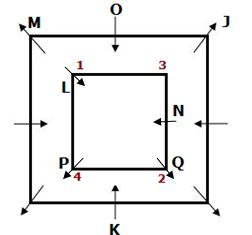Two persons are sitting between P and J, who does not sit in the middle of the sides. Q sits second to the left of N, who is an immediate neighbour of P.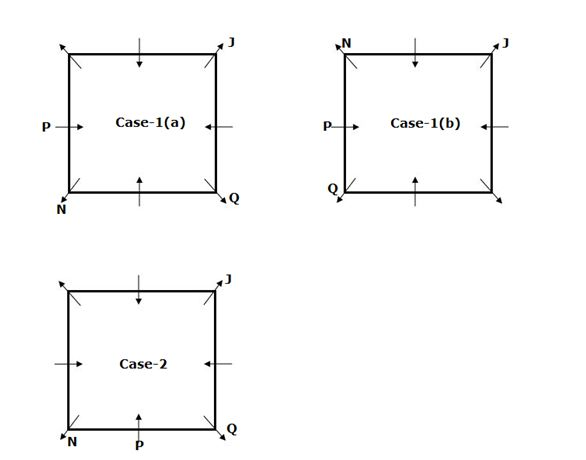L sits second to the right of K. K is not an immediate neighbour of J.

So, Case-2 will be dropped.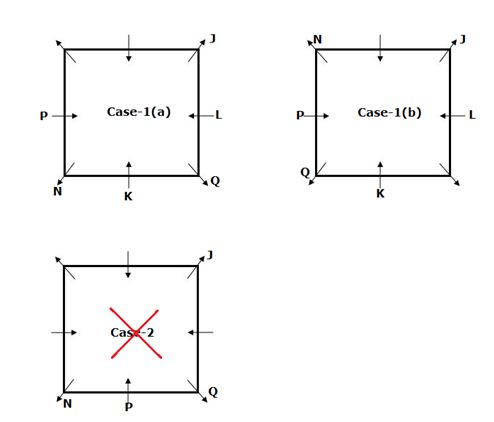M does not sit opposite to K and not an immediate neighbour of L.

So, Case-1(b) will be dropped.

Throws:

1. L throws the dies and the sum is 7.
2. P throws the dies and the sum is 4.
3. Q throws the dies and the sum is 6.
4. N throws the dies and the sum is 10.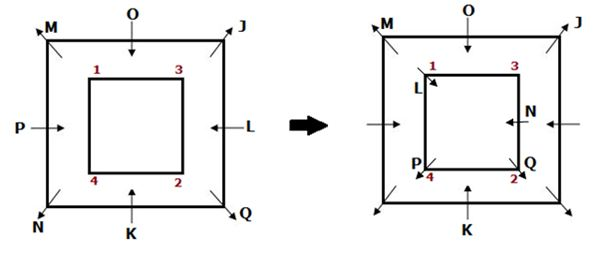Direction (6-10):

I. A≥S –> A>D≥K>S –> False

II. D>W –> D≥K=N≥W –> False

III. W=D –> D≥K=N≥W –> False

But, D≥W is true.

I. G>F –> G≥R<F –> False

II. D≤G –> D=E≤R≤G –> True

III. P<D –> P≤R≥E=D –> False

I. H<J –> H=C≤M<J –> True

II. N≥K –> N≥H=C=K –> True

III. M>N  –> M≥C=H≤N –> False

I. M≥J –>M>K=U≤J –> False

II. V>U –> V≥M>K=U –> True

III. U≤V –> V≥M>K=U –> False

I. R=H –> R=W≥D>H –> False

II. D≤T –> D≤W=R<T –> False

III. J>H –> J≥R=W≥D>H –> True

Daily Practice Test Schedule | Good Luck

 Topic Daily Publishing Time Daily News Papers & Editorials 8.00 AM Current Affairs Quiz 9.00 AM Current Affairs Quiz (Hindi) 9.30 AM IBPS Clerk Prelims – Reasoning 10.00 AM IBPS Clerk Prelims – Reasoning (Hindi) 10.30 AM IBPS Clerk Prelims – Quantitative Aptitude 11.00 AM IBPS Clerk Prelims – Quantitative Aptitude (Hindi) 11.30 AM Vocabulary (Based on The Hindu) 12.00 PM IBPS Clerk Prelims – English Language 1.00 PM SSC Practice Questions (Reasoning/Quantitative aptitude) 2.00 PM IBPS PO/Clerk – GK Questions 3.00 PM SSC Practice Questions (English/General Knowledge) 4.00 PM Daily Current Affairs Updates 5.00 PM IBPS PO Mains – Reasoning 6.00 PM IBPS PO Mains – Quantitative Aptitude 7.00 PM IBPS PO Mains – English Language 8.00 PM